or# Mensuration NCERT Solutions : Class 8 Maths

## NCERT Solutions for Class 8 Maths : MensurationFilter
Filters :
Classes
•
•
•
•
•
•
•
Chapters
•
•
•
•
•
•
•
•
•
•
• 5 More
Exercises
•
•
•
•
•

### NCERT Class 8 | MENSURATION | Exercise 03 | Question No. 08

The lateral surface area of a hollow cylinder is `4224cm^2.` It is cut along its height and formed a rectangular sheet of width `33 cm.` Find the perimeter of rectangular sheet?Doubtnut one of the best online education platform provides free NCERT Solutions of Maths for Class 8 Mensuration which are solved by our maths experts as per the NCERT (CBSE) guidelines. We provide the solutions as video solutions in which concepts are also explained along with answer which will help students while learning , preparing for exams and in doing homework. These Solutions will help to revise complete syllabus and score more marks in examinations.

Get here free, accurate and comprehensive NCERT Solutions for Class 8 Maths Mensuration which have been reviewed by our maths counsellors as per the latest edition following up the CBSE guidelines. We provide video solutions in which solutions to all the questions of NCERT Class 8 Maths textbook are explained in step by step and detailed way.

## The Topics of the Chapter Mensuration are :

REVIEW

SURFACE AREAS OF A CUBOID AND A CUBE

CUBE

SURFACE AREA OF A RIGHT CIRCULAR CYLINDER

SURFACE AREA OF THE WALLS OF A ROOM

AREA OF A TRAPEZIUM

AREA OF A POLYGON

INTRODUCTION

FORMULA FOR FINDING THE VOLUME OF A CUBOID

VOLUME OF A CYLINDER

VOLUME OF A CUBE

It contains these exercises along with solved examples. All the exercises are solved in the video. Select the exercise to view the solutions exercisewise of the Chapter Mensuration:

## NCERT Solutions Class 8 Maths Chapter Mensuration Exercises:

We have covered all the exercises and also Solved examples in the videos. Along with the practise exercise Students should also practise solved examples to clear the concepts of the Mensuration. If incase you have any doubt you can watch the solutions for the given questions.Watch the solutions for the given questions in which it is also explained in the video steps to solve the questions along with answers.

# NCERT Class 8 Maths Solutions Chapter 11 Mensuration

Getting the right information on Mensuration for the students is very important.  In this chapter, new concepts like the area of a trapezium, area of general quadrilaterals, area of special quadrilaterals, area of a polygon, solid shapes, etc. have been discussed in the best way. Finding all the solutions have been made possible by Doubtnut with the help of its online video tutorials. So, this helps the students to get the right idea on how to clear all their queries in the right manner. It is also possible to get all the NCERT Class 8 Maths Solutions Chapter 11 from Doubtnut at zero cost that proves to be an added advantage.

NCERT textbooks are the core books for the students gaining education under CBSE board.  Students are able to clear the basic concepts with the NCERT maths books; however, it does not prove to be useful in solving difficult questions in the exams. Therefore, in order to get the right and clear solutions of the different tricky questions, they try to look forward to the different reference books that leads to waste of time and money. Moreover, student has to attend tuitions to resolve his/her doubts. To overcome this issue, Doubtnut provides free video solutions in easy to understand language for NCERT books. The doubt clearance by doubtnut experts prove to be a boon for students in exams.

In order to provide the perfect and clear idea on the different topics and that too in the easiest manner, a team of experienced teachers at Doubtnut has come up with the simple, yet effective online study material. This helps in proving to be quite useful for the students where they can get step-by-step instructions on how to work on the different questions of Mensuration. It follows up the CBSE guidelines for which the students get the perfect idea on how to attempt the different questions easily. Being the best online education platform, it is also free for which students do not have to pay any fees for it. So, it helps them to get the right idea on how to find the right solutions to different problems without having to look elsewhere.

## Topics and subtopics included in NCERT Class 8 Maths Solutions Chapter 11 Mensuration

### Introduction

It has been seen that in case of a closed plane figure, the perimeter happens to be the distance around its boundary and the region covered by it is called area. We have already learned how to find the area and perimeter of rectangles, triangle, circles and so on. It has already been discussed as to how to find the areas of borders or pathways when it comes to the rectangular shapes. Well, in this chapter, students would be able to come up with the perfect solution related to perimeter as well as others like finding the area of quadrilaterals. It would also be possible to get important information on how to calculate the surface area as well as volumes of cuboid, cube, and cylinder.

### Area of Trapezium

If you wish to know the area of a trapezium, then it is important to get the right knowledge on both the length as well as perpendicular distances between the two parallel sides. The perpendicular distance and half the sum product of the parallel sides’ length between them help in getting the area of a trapezium.

### Area of a General Quadrilateral

It is possible to split a general quadrilateral into two triangles. This can be done by drawing one of its diagonals. In order to get a good idea, let’s have a look at it.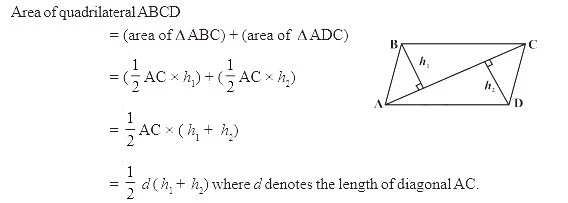In order to find a formula for the area of a rhombus, the same method of triangulation is used. In the below figure, ABCD is a rhombus. So, it is important to note that the diagonals are perpendicular bisectors of each other.

Area of Rhombus ABCD = (Area of ACD ) + (Area of ABC)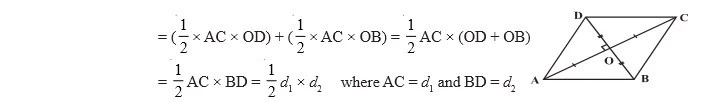### Area of a Polygon

A quadrilateral is split into triangles to find its area. It should be mentioned that the same methods can be utilized when it comes to finding the area of a polygon. In the below figure we construct two diagonals AC and AD where the pentagon ABCDE has been divided into three parts.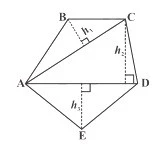So, the area of pentagon ABCDE = area of Δ ABC + area of Δ ACD + area of Δ AED.

### Solid Shapes

In our earlier chapters, we have learned about two dimensional figures where it can be identified as the faces of three-dimensional shapes. Let’s have a clear idea about it: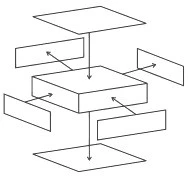In Cuboidal box, all six faces are rectangular, and opposites faces are identical. So, there are three pairs of identical faces.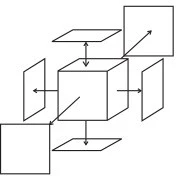In Cubical box, all six faces are squares and identical.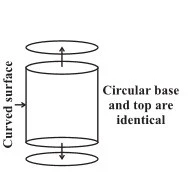In Cylindrical Box, one curved surface and two circular faces which are identical.

### Surface Area of Cube, Cuboid, and Cylinder

When it comes to the surface area of a solid, it means the sum of the areas of all its faces.  In the case of cuboid, it is to be kept in mind that it has got three pairs of identical faces. So, in the same way, it is very important to understand as to how you can get the surface area of a cube and cylinder as well.

### The volume of Cube, Cuboid, and Cylinder

Volume is the amount of space which is occupied by a three-dimensional object. In order to find the area of a region, we use square units.

1 cubic cm = 1 cm × 1 cm × 1 cm = 1 cm3

= 10 mm × 10 mm × 10 mm = mm3

1 cubic m = 1 m × 1 m × 1 m = 1 m3 = cm3

1 cubic mm = 1 mm × 1 mm × 1 mm = 1 mm3

= 0.1 cm × 0.1 cm × 0.1 cm = cm3

Volume of a cube = l x l x l = l3

The volume of a cuboid = area of base × height

= l × b × h = lbh

The volume of cylinder = area of base × height

= πr2 × h = πr2h

### Volume and Capacity

When it comes to these two terms, there is not much difference:

The amount of space occupied by an object is called Volume where the quantity that the container holds is called Capacity.

Find the height of a cuboid whose volume is 275 cm3 and the base area is 25 cm2.

Solution:  Volume of a cuboid = Base area × Height

Hence the height of the cuboid = Volume of cuboid/Base area

= 275/25

= 11 cm

Height of the cuboid is 11 cm.

## Importance of Doubtnut for the students

The Class 8 Maths NCERT Solutions provided by doubtnut help a lot to the students in understanding the mathematical concepts swiftly. There are several other advantages of learning maths from doubtnut that include:

• Use of simple language: All the lessons or topics are explained by the expert tutors of Doubtnut in simple language. This helps a lot to get the perfect idea on how to clear the queries of the students effectively.  Students appearing for competitive exams are also benefited from Doubtnut’s video tutorials that prove to be of much use to them. Students can learn it from the comfort of their place which proves to be another advantage to them.
• Lots of exercises and examples included:  A lot of examples and exercises have been included where students do not find it difficult at all to solve even the most complicated questions. So, this helps to boost their confidence where they do not have to worry for any sort of reasons. Being the 24/7 open learning platform, you can learn and study anytime from doubtnut.
• Get previous year question papers: Another advantage that students are able to get is the previous year solved question papers solved. This really helps a lot the students to get a good idea regarding questions of different patterns that are asked in the exams.  Therefore, students who are really serious about getting good marks in maths should opt for the most useful online video tutorials provided by Doubtnut.